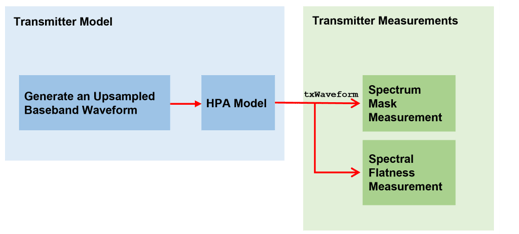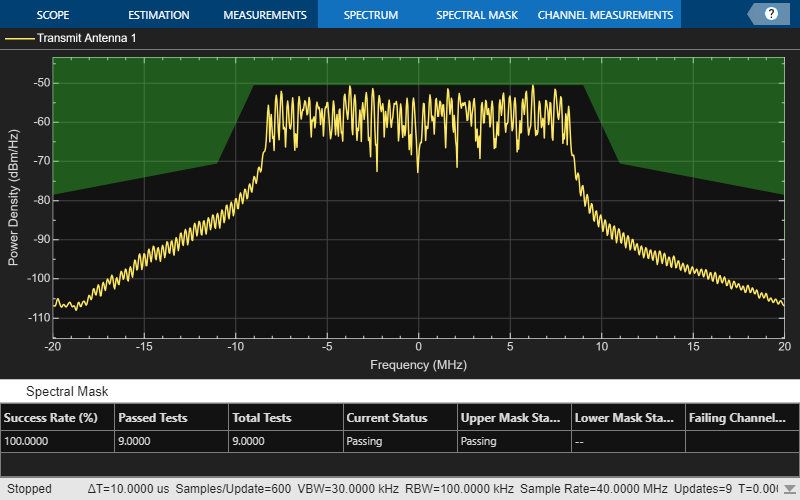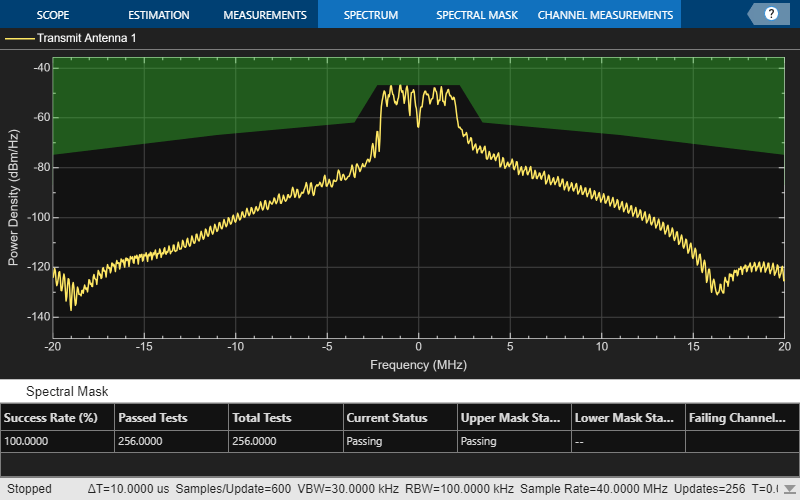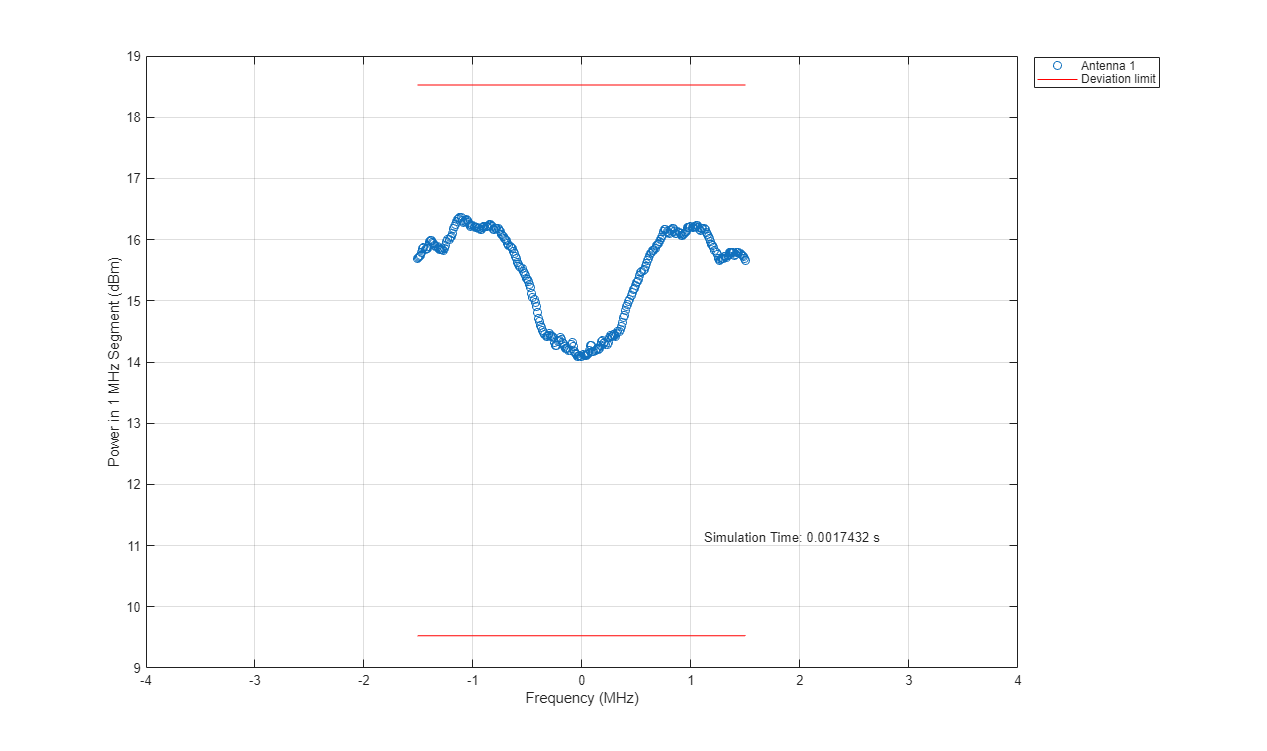# 802.11ba WUR Waveform Generation and Analysis

This example shows how to generate IEEE® 802.11ba™ wake-up radio (WUR) packet waveforms. The example also demonstrates how to measure the transmit spectrum mask and spectral flatness.

### Introduction

The 802.11ba draft standard [ 1 ], referred to as wake-up radio (WUR), defines a mechanism to enable IEEE 802.11 stations (STAs) to operate at extremely low power consumption and to react to incoming traffic with low latency through a wake-up signal. This standard defines two types of WUR PPDU format.

• WUR Basic PPDU with 20 MHz channel bandwidth (one subchannel)

• WUR FDMA PPDU with 40 MHz (two subchannels) or 80 MHz (four subchannels) contiguous channel bandwidths

Each 20 MHz subchannel can transmit data for a single user. For each 20 MHz subchannel, the WUR physical layer (PHY) provides support for data rates of 62.5 kb/s and 250 kb/s, indicated as low data rate (LDR) and high data rate (HDR), respectively. Additionally, Annex AC of [ 1 ] provides three examples of symbol sequences and cyclic shift durations (CSDs) at specified data rates.

This example generates 5 WUR packets with a 20 MHz channel bandwidth and 10 microsecond gaps between each of the packets. The example oversamples the waveform using a larger inverse fast Fourier transform (IFFT) length than required for the nominal baseband rate and does not perform spectral filtering. The example then models the effects of a high-power amplifier (HPA), which introduces in-band distortion and spectral regrowth. After HPA modelling, the example extracts the non-WUR portion of the WUR PHY preamble, comprising the L-STF, L-LTF, L-SIG, BPSK-Mark1, and BPSK-Mark2 fields, and the WUR portion, comprising the WUR-Sync and WUR-Data fields, from the generated waveform. The example then performs two transmit spectrum mask measurements for the non-WUR portion of WUR PHY preamble and WUR portion, respectively, and measures the spectral flatness for the WUR portion. This figure shows the workflow contained in this example.### Simulation Setup

Configure the example to generate 5 WUR packets with a 10 microsecond idle period between each packet.

```numPackets = 5; idleTime = 10; % In microseconds ```

### 802.11ba WUR Waveform Configuration and Generation

Create a WUR configuration object for a 20 MHz transmission.

```numSubchannels = 1; % Number of subchannels cfgWUR = wlanWURConfig(numSubchannels); % Create WUR packet configuration cfgWUR.NumTransmitAntennas = 1; % Number of transmit antennas fs = wlanSampleRate(cfgWUR); % Get the nominal baseband sample rate osf = 2; % Oversampling factor ```

Parameterize the transmission by setting WUR configuration object properties for each user. The waveform generator function uses only the first `cfgWUR.NumUsers` elements of the `cfgWUR` cell array to generate the corresponding WUR packets.

```psdu = cell(1,cfgWUR.NumUsers); psduLength = [5,10,15,20]; % Bytes, 1 to 22 inclusive for each 20 MHz subchannel dataRate = {'LDR','HDR','LDR','HDR'}; symDesign = {'Example1','Example2','Example1','Example2'}; rng(0); % Set random state for i = 1:cfgWUR.NumUsers cfgWUR.Subchannel{i}.PSDULength = psduLength(i); psdu{i} = randi([0 1],psduLength(i)*8,1,'int8'); cfgWUR.Subchannel{i}.DataRate = dataRate{i}; cfgWUR.Subchannel{i}.SymbolDesign = symDesign{i}; end ```

Generate the WUR waveform for the specified bits and configuration by using the `wlanWaveformGenerator` function, setting the number of packets, idle time between each packet, and oversampling factor.

```txWaveform = wlanWaveformGenerator(psdu,cfgWUR,'NumPackets',numPackets,'IdleTime',idleTime*1e-6,'OversamplingFactor',osf); ```

The HPA introduces nonlinear behaviour in the form of in-band distortion and spectral regrowth. This example simulates the power amplifiers by using the Rapp model, which introduces AM/AM distortion [ 2 ]. Model the amplifier by using the `comm.MemorylessNonlinearity` object, and configure reduced distortion by specifying a back-off, `hpaBackoff`, such that the amplifier operates below its saturation point.

```pSaturation = 25; % Saturation power of a power amplifier in dBm hpaBackoff = 15; % Power amplifier backoff in dB nonLinearity = comm.MemorylessNonlinearity; nonLinearity.Method = 'Rapp model'; nonLinearity.Smoothness = 3; % p parameter nonLinearity.LinearGain = -hpaBackoff; nonLinearity.OutputSaturationLevel = db2mag(pSaturation-30); ```

Apply the HPA model to the waveform.

```txWaveform = nonLinearity(txWaveform); ```

Add thermal noise to each transmit antenna by using the `comm.ThermalNoise` object with a noise figure of 6 dB [ 3 ].

```thNoise = comm.ThermalNoise('NoiseMethod','Noise Figure','SampleRate',fs*osf,'NoiseFigure',6); for i = 1:cfgWUR.NumTransmitAntennas txWaveform(:,i) = thNoise(txWaveform(:,i)); end ```

### Transmit Spectrum Mask and Spectral Flatness Measurement

Get indices for accessing WUR fields within the time-domain packet.

```ind = wlanFieldIndices(cfgWUR,'OversamplingFactor',osf); % Get the number of samples in the idle time. numIdle = osf*fs*idleTime*1e-6; % Define the length of a WUR packet, in samples. pktLength = double(max(ind.WURData(:,2)))+numIdle; % Define the length of non-WUR portion of the WUR PHY preamble, composed of the % L-STF, L-LTF, L-SIG, BPSK-Mark1 and BPSK-Mark2 fields, in samples. idxPreamble = zeros(ind.BPSKMark2(2),numPackets,'uint32'); % Define the length of the WUR-Sync and WUR-Data fields, in samples. idxWUR = zeros(max(ind.WURData(:,2))-ind.WURSync(1,1)+1,numPackets,'uint32'); ```

Extract the non-WUR portion of the WUR PHY preamble and WUR portion of each packet within the waveform.

```pktOffset = 0; % Start at first sample (no offset) for i = 1:numPackets % Indices of WUR preamble fields idxPreamble(:,i) = pktOffset+(1:ind.BPSKMark2(2)); % Indices of WUR-Sync and WUR-Data fields idxWUR(:,i) = pktOffset+(ind.WURSync(1,1):max(ind.WURData(:,2))); % Packet offset to generate the end index of each packet pktOffset = i*pktLength; end preamFields = txWaveform(idxPreamble(:),:); wurFields = txWaveform(idxWUR(:),:); ```

Measure the transmit spectrum mask of the non-WUR portion of the WUR PHY preamble, as specified in Section 30.3.12.1 of [ 1 ].

```if numPackets>0 helperSpectralMaskTest(preamFields,fs,osf); end ```
``` Spectrum mask passed ```Based on the values of limits (in dBr) relative to maximum spectral density of the signal and corresponding frequency offsets (in MHz) provided in Section 30.3.12.1 of [ 1 ], define the transmit spectrum masks of the WUR-Sync and WUR-Data fields for WUR basic and FDMA PPDUs are.

```switch numSubchannels case 1 % WUR Basic PPDU dBrLimits = [-40 -40 -28 -20 -15 0 ... 0 -15 -20 -28 -40 -40]; fLimits = [-Inf -30 -20 -11 -3.5 -2.25 ... 2.25 3.5 11 20 30 Inf]; case 2 % 40 MHz WUR FDMA PPDU dBrLimits = [-40 -40 -28 -20 -15 0 0 -15 -20 ... -20 -15 0 0 -15 -20 -28 -40 -40]; fLimits = [-Inf -60 -40 -21 -13.5 -12.25 -7.75 -6.5 -1 ... 1 6.5 7.75 12.25 13.5 21 40 60 Inf]; otherwise % 80 MHz WUR FDMA PPDU dBrLimits = [-40 -40 -28 -20 -15 0 0 -15 -20 -20 -15 0 0 -15 -20 ... -20 -15 0 0 -15 -20 -20 -15 0 0 -15 -20 -28 -40 -40]; fLimits = [-Inf -120 -80 -41 -33.5 -32.25 -27.75 -26.5 -21 -19 -13.5 -12.25 -7.75 -6.5 -1 ... 1 6.5 7.75 12.25 13.5 19 21 26.5 27.75 32.25 33.5 41 80 120 Inf]; end ```

Measure the transmit spectrum mask and spectral flatness of the WUR-Sync and WUR-Data fields, as specified in Section 30.3.12.1 and Section 30.3.12.2 of [ 1 ]. The `wurTxSpectralFlatnessMeasurement` function measures the spectral flatness by comparing the power in any contiguous 1 MHz segment within the center 4 MHz of each 20 MHz channel to the total transmitted power in the center 4 MHz and measures the transmitted power in the manner described in Section 5.4.3.2.1 of [ 4 ] for equipment with continuous and noncontinuous transmissions.

```if numPackets>0 helperSpectralMaskTest(wurFields,fs,osf,dBrLimits,fLimits); isPassed = wurTxSpectralFlatnessMeasurement(wurFields,fs,osf); if isPassed fprintf(' Spectral flatness passed\n'); else fprintf(' Spectral flatness failed\n'); end end ```
``` Spectrum mask passed Spectral flatness passed ```### Conclusion and Further Exploration

This example shows how to generate WUR waveforms as specified in the IEEE 802.11ba draft standard, and measure these quantities.

• Spectral flatness

The HPA model introduces significant in-band distortion and spectral regrowth, which is visible in the spectral mask plot. Try increasing the value of the HPA backoff and observe lower out-of-band emissions. The various patterns of ripple and deviation limits in the spectral flatness measurement is due to the different Multi-Carrier On-Off Keying (MC-OOK) On symbol designs, such as MC-OOK On symbol sequences and CSD values applied. The spectral flatness test will fail for a 20 MHz subchannel if the subchannel in the generated waveform is configured with symbol design and data rate set to `'Example3'` and `'HDR'`, respectively. Try using a different MC-OOK On symbol design and observe the impact on the spectral flatness.

### References

1. IEEE P802.11ba™/D8.0 Draft Standard for Information technology - Telecommunications and information exchange between systems Local and metropolitan area networks - Specific requirements - Amendment 3: Wake-Up Radio Operation.

2. R. Porat, et al. TGax Evaluation Methodology, IEEE 11/14-0571r12. 2016-01-21.

3. Perahia, E., and R. Stacey. Next Generation Wireless LANs: 802.11n and 802.11ac. 2nd Edition. United Kingdom: Cambridge University Press, 2013. Archambault, Jerry, and Shravan Surineni. "IEEE 802.11 spectral measurements using vector signal analyzers." RF Design 27.6 (2004): 38-49.

4. ETSI EN 300 328 V2.1.1. Wideband transmission systems; Data transmission equipment operating in the 2,4 GHz ISM band and using wide band modulation techniques; Harmonised Standard covering the essential of article 3.2 of Directive 2014/53EU. 2016-11.